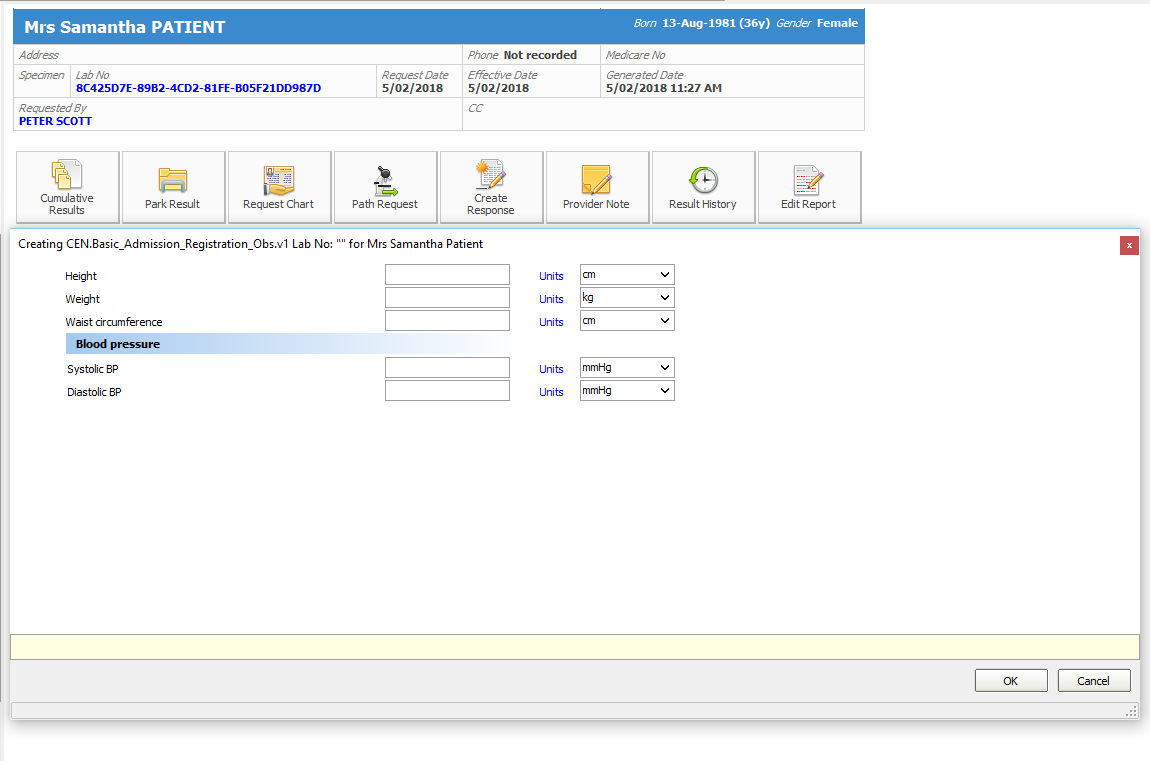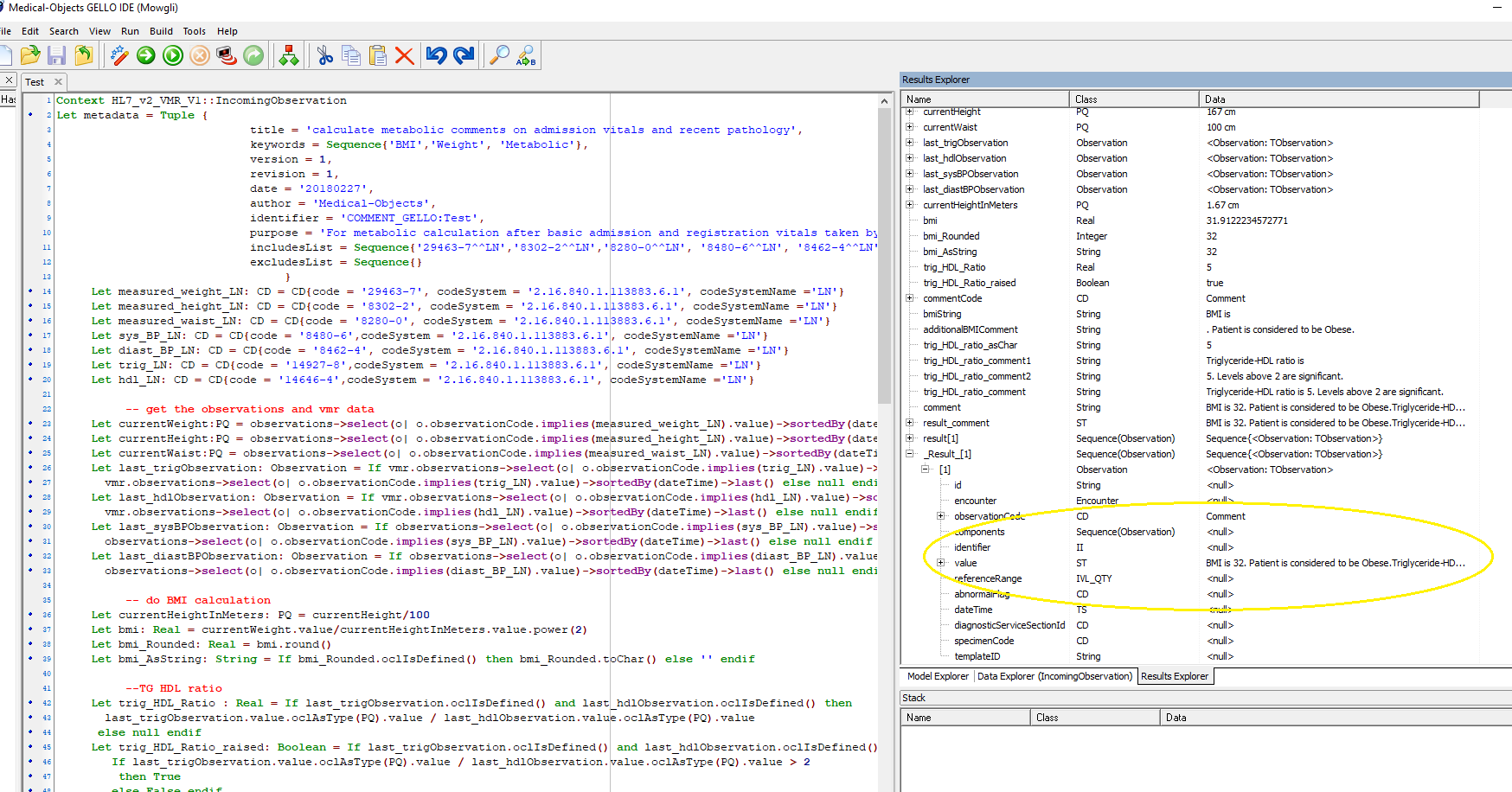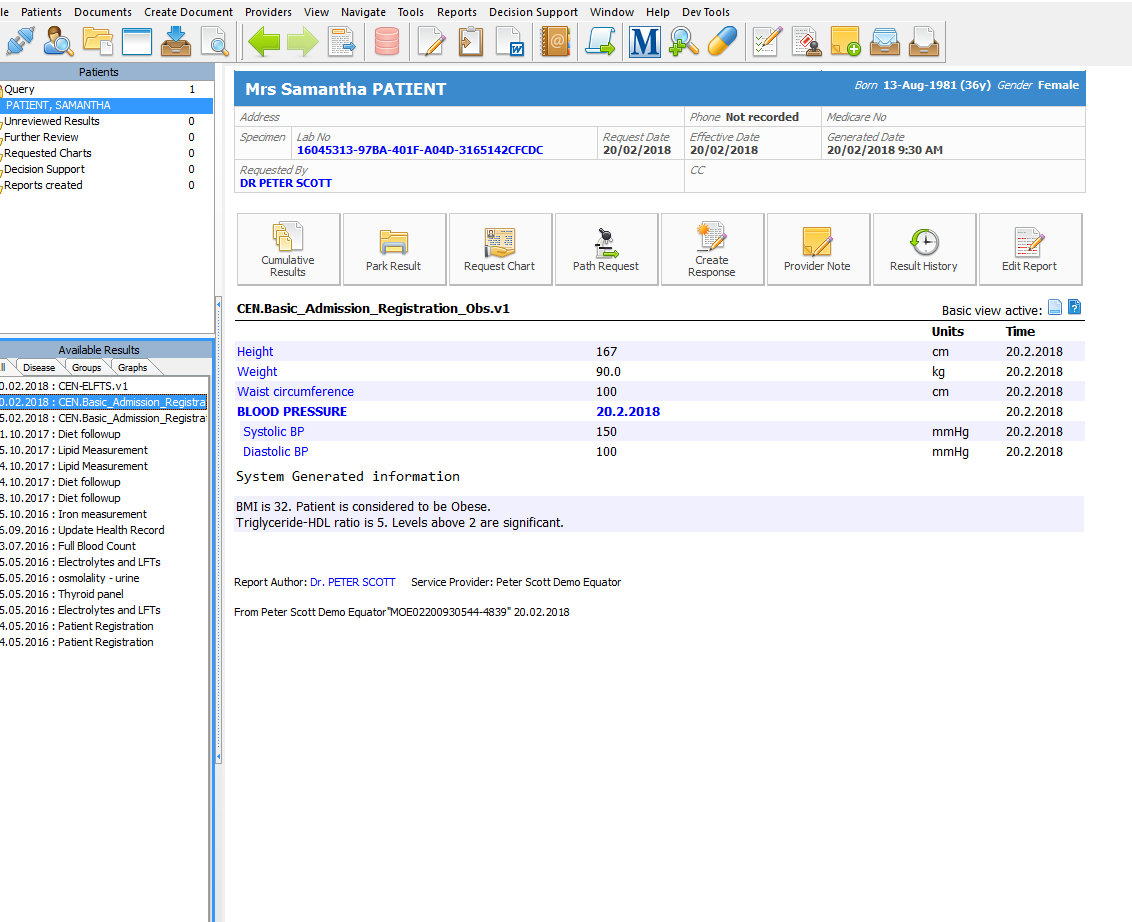##### Page tree

Should you require any more information or have encountered a problem, please call the support helpdesk on (07) 5456 6000.

Go to start of banner

# Comment gello

GELLO can have the context of an IncomingObservation, for example a pathology result. Metadata within the GELLO can be set to specify LOINC codes for a host system to watch for and if present, run the GELLO.

This worked example will demonstrate using screen shots from the Medical Objects Explorer software. It will show some GELLO that looks for archetyped clinical patient data of height , weight, waist circumference and Blood pressure, such as might be obtained when a patient presents to a medical clinic. The code will take this data, and also look for recent blood tests results; and run its logic as to whether certain conditions are present such as being overweight or having abnormal metabolic profiles. We will use BMI and Triglyceride/HDL ratio. The result of the GELLO is another observation that presents in the UI as System Generated information, below the displayed Observation that has triggered the Comment GELLO.

The context is:

`     Context HL7_v2_VMR_V1::IncomingObservation`

Metadata is a tuple  we create as a local variable and is customisable. MO Explorer knows to look for this when the GELLO is uploaded to the server it uses and stores the Comment GELLO for clinical use.

`     Let metadata = Tuple {                            title = 'calculate metabolic comments on admission vitals and recent pathology',                            keywords = Sequence{'BMI','Weight', 'Metabolic'},                            version = 1,                            revision = 16,                            date = '20180227',                            author = 'Medical-Objects',                            identifier = 'COMMENT_GELLO:CALCULATE_Metabolic_Comments',                            purpose = 'For metabolic calculation after basic admission and registration vitals taken by nursing staff',                            includesList = Sequence{'29463-7^^LN','8302-2^^LN','8280-0^^LN', '8480-6^^LN', '8462-4^^LN'},                            excludesList = Sequence{}                                 }`

Now we can set up come codes for the retrievable data:

`     Let measured_weight_LN: CD = CD{code = '29463-7', codeSystem = '2.16.840.1.113883.6.1', codeSystemName ='LN'}     Let measured_height_LN: CD = CD{code = '8302-2', codeSystem = '2.16.840.1.113883.6.1', codeSystemName ='LN'}     Let measured_waist_LN: CD = CD{code = '8280-0', codeSystem = '2.16.840.1.113883.6.1', codeSystemName ='LN'}     Let sys_BP_LN: CD = CD{code = '8480-6', codeSystem = '2.16.840.1.113883.6.1', codeSystemName ='LN'}     Let diast_BP_LN: CD = CD{code = '8462-4', codeSystem = '2.16.840.1.113883.6.1', codeSystemName ='LN'}`

These will be the codes that the archetyped data is mapped to.

Add the required lab data LOINC codes:

`     Let trig_LN: CD = CD{code = '14927-8',codeSystem = '2.16.840.1.113883.6.1', codeSystemName ='LN'}     Let hdl_LN: CD = CD{code = '14646-4',codeSystem = '2.16.840.1.113883.6.1', codeSystemName ='LN'}`

Now we get the observation data and the vmr data:

`     -- get the observations and vmr data     Let currentWeight:PQ = observations->select(o| o.observationCode.implies(measured_weight_LN).value)->sortedBy(dateTime)->last().value.oclAsType(PQ)     Let currentHeight:PQ = observations->select(o| o.observationCode.implies(measured_height_LN).value)->sortedBy(dateTime)->last().value.oclAsType(PQ)     Let currentWaist:PQ = observations->select(o| o.observationCode.implies(measured_waist_LN).value)->sortedBy(dateTime)->last().value.oclAsType(PQ)     Let last_trigObservation: Observation = If vmr.observations->select(o| o.observationCode.implies(trig_LN).value)->sortedBy(dateTime)->last().oclIsDefined() then       vmr.observations->select(o| o.observationCode.implies(trig_LN).value)->sortedBy(dateTime)->last() else null endif     Let last_hdlObservation: Observation = If vmr.observations->select(o| o.observationCode.implies(hdl_LN).value)->sortedBy(dateTime)->last().oclIsDefined() then       vmr.observations->select(o| o.observationCode.implies(hdl_LN).value)->sortedBy(dateTime)->last() else null endif     Let last_sysBPObservation: Observation = If observations->select(o| o.observationCode.implies(sys_BP_LN).value)->sortedBy(dateTime)->last().oclIsDefined() then       observations->select(o| o.observationCode.implies(sys_BP_LN).value)->sortedBy(dateTime)->last() else null endif     Let last_diastBPObservation: Observation = If observations->select(o| o.observationCode.implies(diast_BP_LN).value)->sortedBy(dateTime)->last().oclIsDefined() then       observations->select(o| o.observationCode.implies(diast_BP_LN).value)->sortedBy(dateTime)->last() else null endif`

Notice that for the data obtained from the IncomingObservation ie the measured height, weight, waist circumference, systolic blood pressure and diastolic blood pressure; we don't need to say 'vmr.observations', but when we are looking outside the context of the incoming observation, we do- e.g. for recent pathology. This code keeps the convention of searching for Observations, then ordering them by dateTime, then getting the last Observation. This is probably unnecessary for the archetyped clinical data, but it is possible to repeat a blood pressure reading if high ; so it is retained.

Do a BMI calculation: ( we know the units  will be cm for height and kg for weight.

`     -- do BMI calculation     Let currentHeightInMeters: PQ = currentHeight/100     Let bmi: Real = currentWeight.value/currentHeightInMeters.value.power(2)     Let bmi_Rounded: Real = bmi.round()     Let bmi_AsString: String = If bmi_Rounded.oclIsDefined() then bmi_Rounded.toChar() else '' endif`

Do a triglyceride / HDL ratio:

`     --TG HDL ratio     Let trig_HDL_Ratio : Real = If last_trigObservation.oclIsDefined() and last_hdlObservation.oclIsDefined() then       last_trigObservation.value.oclAsType(PQ).value / last_hdlObservation.value.oclAsType(PQ).value      else null endif     Let trig_HDL_Ratio_raised: Boolean = If last_trigObservation.oclIsDefined() and last_hdlObservation.oclIsDefined() then        If last_trigObservation.value.oclAsType(PQ).value / last_hdlObservation.value.oclAsType(PQ).value > 2         then True        else False endif       else False endif`

`     -- prepare comments     Let commentCode:CD =CD{code = '8251-1', codeSystem = '2.16.840.1.113883.6.1', codeSystemName ='LN', displayName = ST{value='Comment'}}     Let bmiString: String = 'BMI is '     Let additionalBMIComment: String = If bmi.oclIsDefined() then        If bmi <18.6 then '. Patient is considered to be Underweight.' else        If bmi >25 and bmi < 30 then '. Patient is considered to be Overweight.' else        If bmi >29.9 then '. Patient is considered to be Obese.' else         ''       endif endif endif else '' endif     Let trig_HDL_ratio_asChar: String = If trig_HDL_Ratio.oclIsDefined() then trig_HDL_Ratio.toChar() else '' endif`
`     Let trig_HDL_ratio_comment1: String = If trig_HDL_Ratio_raised then`
`        '\nTriglyceride-HDL ratio is ' else '' endif     Let trig_HDL_ratio_comment2: String = If trig_HDL_Ratio_raised then trig_HDL_ratio_asChar.concat('. Levels above 2 are significant.')        else '' endif     Let trig_HDL_ratio_comment: String = trig_HDL_ratio_comment1.concat(trig_HDL_ratio_comment2)`
`     Let comment: String = bmiString.concat(bmi_AsString).concat(additionalBMIComment).concat(trig_HDL_ratio_comment)`

Produce the result:

`     -- result      Let result_comment:ST = ST{value=comment}     Let result:Sequence(Observation)                = Sequence{Observation{                                       value = result_comment,                                       observationCode = commentCode                                       }                           }     result`

OK! So here's the input screen in MO Explorer, for the archetype that becomes the Incoming Observation that the GELLO is watching for the LOINC codes for (Comment GELLO just as easily runs against HL7 lab data Observations):Here's what happens when we run the gello explicitly against this patient's data:Here's the UI of the Observation showing the comment as a string under 'System Generated information':• No labels